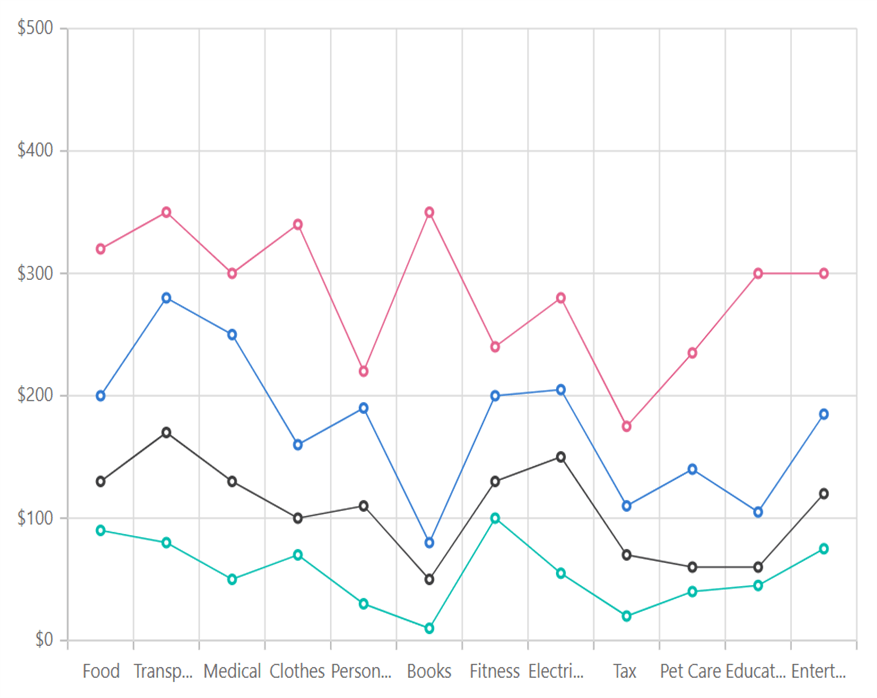Search results

# Stacked Line in Blazor Chart component

## Stacked

Type of line chart where lines of the data don’t get overlapped because they will be cumulative at each point. To render a stacked line series, use series `Type` as `StackingLine`.

stacked-line.razor
``````@using Syncfusion.Blazor.Charts

<SfChart Title="Family Expense for Month">
<ChartPrimaryXAxis ValueType="Syncfusion.Blazor.Charts.ValueType.Category" Interval="1">
</ChartPrimaryXAxis>

<ChartPrimaryYAxis Title="Expense" Interval="100" LabelFormat="\${value}">
</ChartPrimaryYAxis>

<ChartArea>
<ChartAreaBorder Width="0"></ChartAreaBorder>
</ChartArea>

<ChartSeriesCollection>
<ChartSeries XName="X" Width="2" DashArray="5,1" DataSource="@ExpenseReports"
YName="Y" Type="ChartSeriesType.StackingLine">
<ChartMarker Visible="true">
</ChartMarker>
</ChartSeries>
<ChartSeries XName="X" Width="2" DashArray="5,1" DataSource="@ExpenseReports"
YName="Y1" Type="ChartSeriesType.StackingLine">
<ChartMarker Visible="true">
</ChartMarker>
</ChartSeries>
<ChartSeries XName="X" Width="2" DashArray="5,1" DataSource="@ExpenseReports"
YName="Y2" Type="ChartSeriesType.StackingLine">
<ChartMarker Visible="true">
</ChartMarker>
</ChartSeries>
<ChartSeries XName="X" Width="2" DashArray="5,1" DataSource="@ExpenseReports"
YName="Y3" Type="ChartSeriesType.StackingLine">
<ChartMarker Visible="true">
</ChartMarker>
</ChartSeries>
</ChartSeriesCollection>
</SfChart>

@code{
public class ChartData
{
public string X { get; set; }
public double Y { get; set; }
public double Y1 { get; set; }
public double Y2 { get; set; }
public double Y3 { get; set; }
}
public List<ChartData> ExpenseReports = new List<ChartData>
{
new ChartData { X = "Food" , Y = 90, Y1 = 40 , Y2= 70, Y3= 120},
new ChartData { X = "Transport", Y = 80, Y1 = 90, Y2= 110, Y3= 70 },
new ChartData { X = "Medical",Y = 50, Y1 = 80, Y2= 120, Y3= 50 },
new ChartData { X = "Clothes",Y = 70, Y1 = 30, Y2= 60, Y3= 180 },
new ChartData { X = "Personal Care", Y = 30, Y1 = 80, Y2= 80, Y3= 30 },
new ChartData { X = "Books", Y = 10, Y1 = 40, Y2= 30, Y3= 270},
new ChartData { X = "Fitness",Y = 100, Y1 = 30, Y2= 70, Y3= 40 },
new ChartData { X = "Electricity", Y = 55, Y1 = 95, Y2= 55, Y3= 75},
new ChartData { X = "Tax", Y = 20, Y1 = 50, Y2= 40, Y3= 65 },
new ChartData { X = "Pet Care", Y = 40, Y1 = 20, Y2= 80, Y3= 95 },
new ChartData { X = "Education", Y = 45, Y1 = 15, Y2= 45, Y3= 195 },
new ChartData { X = "Entertainment", Y = 75, Y1 = 45, Y2= 65, Y3= 115 }
};
}``````## Customization

You can use the following properties to customize the stacked line series.